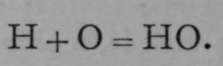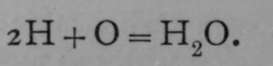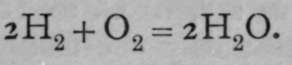Substances consist of two kinds of particles, each of which has been termed an atom by Dalton. But they are in reality different. The smallest particle, or, as Avogadro named it, molecule, of water, consists of three atoms, two of hydrogen and one of oxygen. But hydrogen gas and oxygen gas also consist of molecules, each of them containing two atoms. The act of union of these elements is to be regarded not as a case of combination of atoms, as Dalton supposed, but rather as an exchange of partners; the atom of oxygen leaving the other atom with which it had been combined, and uniting with two atoms of hydrogen, each of which had similarly left its partner. Thus n molecules of oxygen exchange partners with in molecules of hydrogen, and form 2n molecules of water-gas ; but whereas the molecules of oxygen and hydrogen each contain two atoms, those of water-gas contain three. And this explanation is consistent with the facts; for while 2n molecules of hydrogen and n molecules of oxygen, containing together 6n atoms, react to form 2n molecules of water-gas, the latter also contains 6n atoms, for each molecule of water-gas contains three atoms.

Using the symbols H and O for one atom of hydrogen and one atom of oxygen respectively, Dalton's idea of the combination was.

On the assumption that equal volumes of the gases contain equal numbers of atoms, the equation becomes.

Lastly, on Avogadro's hypothesis, that the action is one between molecules of hydrogen and molecules of oxygen, each containing two atoms, the equation is :.

Granting Avogadro's hypothesis, the relative weights of the atoms can be ascertained. For, as oxygen is 16 times as heavy as hydrogen, and as equal volumes of these gases contain equal numbers of molecules, and moreover as each molecule consists of two atoms, it follows that an atom of oxygen is 16 times as heavy as an atom of hydrogen.